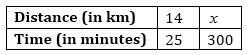# A loaded truck travels 14 km in 25 minutes. If the speed remains the same, how far can it travel in 5 hours?

Solution

Let distance covered in 5 hours be x km.

1 hour = 60 minutes

Therefore, 5 hours = 5×60 = 300 minutesSince the distance travelled by truck is directly proportional to the time taken,

So, 14/25 = a/300

x= 14 x 300 / 25

x = 168 km

The truck travels 168 km in 5 hours.